Next: Collision Parameters Up: Gases and Ideal Gases Previous: Kinetic Gas Theory   Contents   Index

### Maxwell-Boltzmann Distribution

The kinetic energy distribution of electrons, ions and neutrals is very important for characterizing a gas. From statistical arguments and also from experiments the so called Maxwell Boltzmann distribution can be derived (see fig.). This indicates the portion of the gas constituents having a specific velocity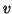in thermal equilibrium (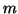denotes the mass of the particles). At www.gs68.de you can plot a Maxwell Boltzmann Distribution online.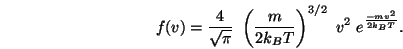(1.7)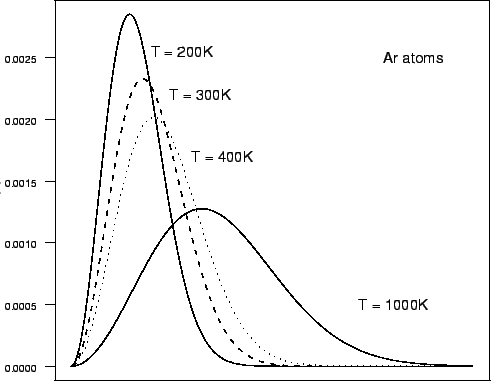The shape of the distribution gets wider for higher temperatures and also the peak decreases. It is possible to calculate a mean speed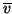which is equal to:(1.8)

The mean speed is always higher than the most probable speed due to the skewness of the distribution. For Argon atoms at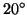C (=295K)= 394m/s.Next: Collision Parameters Up: Gases and Ideal Gases Previous: Kinetic Gas Theory   Contents   Index

back to www.gs68.de